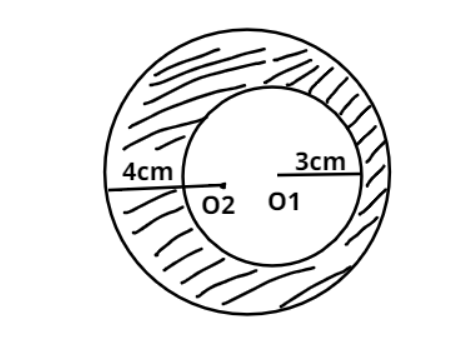Courses
Courses for Kids
Free study material
Free LIVE classes
MoreLIVE
Join Vedantu’s FREE Mastercalss

# From a circular sheet of radius 4 cm, a circle of radius 3 cm is removed. Find the area of the remaining sheet. Take $\left( {\pi = 3.14} \right)$Verified
363.3k+ views
Hint – In this question from the main circular sheet of radius 4 cm, a smaller circle of radius 3 cm is removed. So using the basic formula for area of circle, we can easily remove the smaller circular area from the larger circular area. This concept will give us the required remaining area.Area of the circle of radius r is given as ${\text{A = }}\pi {r^2}$……………………. (1)
Now the radius of the larger circle centered at O2 is having radius r1=4cm……………. (2)
The radius of the smaller circle that is being removed from the larger circle is centered at O1 and has the radius r2=3cm……………………. (3)
Now putting the values in equation (1)
Area of larger circle centered at 02,
${{\text{A}}_2} = \pi {\left( 4 \right)^2} = 16\pi {\text{c}}{{\text{m}}^2}$…………………………… (4)
Now putting the values in equation (1) again
The area of smaller circle centered at O1,
${{\text{A}}_1} = \pi {\left( 3 \right)^2} = 9\pi {\text{c}}{{\text{m}}^2}$……………………… (5)
Now the required remaining area which is shaded in diagram is equal to the difference of larger circle centered at 02 and the area of smaller circle centered at O1.
Thus using equation (4) and (5) we can say that
${\text{Area req = 16}}\pi {\text{ - 9}}\pi \\ \Rightarrow 7\pi \\$
Using $\pi = 3.14$ we get
Area required = $7 \times 3.14 = 21.98{\text{c}}{{\text{m}}^2}$

Note – Whenever we face such types of problems the key concept is to think of the diagrammatic representation using the data provided in the problem. This will give you the actual understanding about which area has to be removed from which area in order to reach the required area.
Last updated date: 01st Oct 2023
Total views: 363.3k
Views today: 4.63k